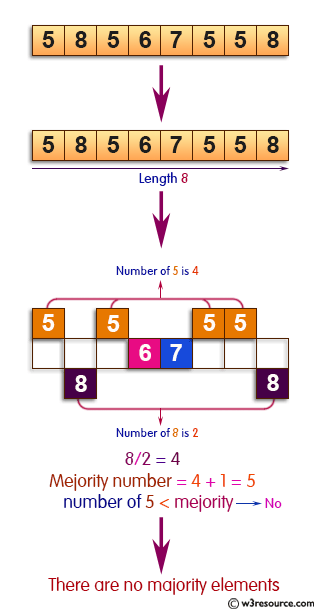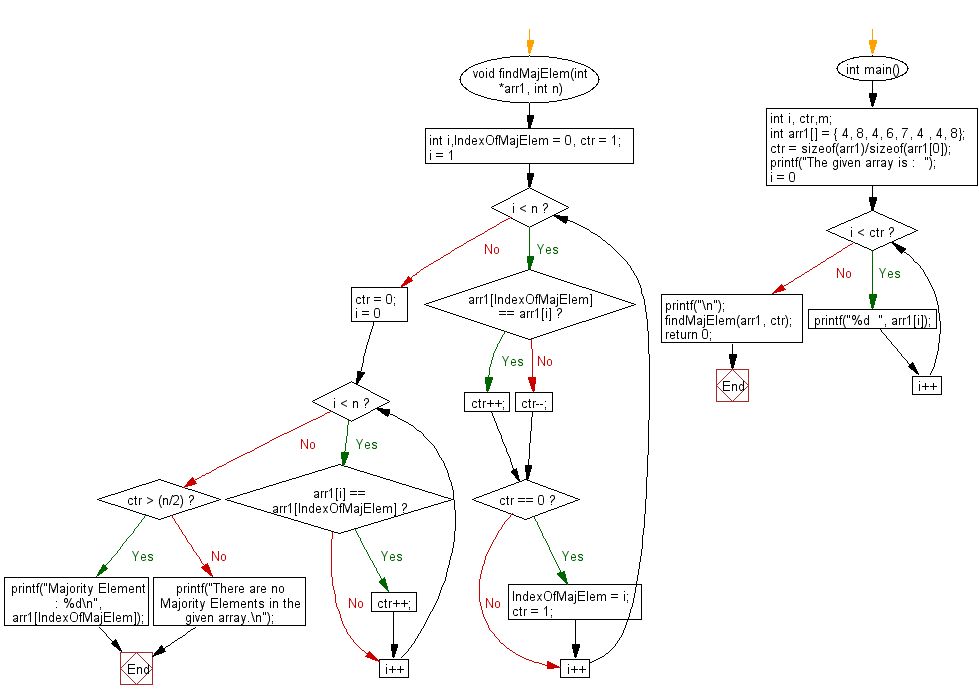﻿ C exercises: Find the majority element of an array - w3resource# C Exercises: Find the majority element of an array

## C Array: Exercise-33 with Solution

Write a program in C to find the majority element of an array.
A majority element in an array A[] of size n is an element that appears more than n/2 times (and hence there is at most one such element).

Pictorial Presentation:Sample Solution:

C Code:

``````#include <stdio.h>

void findMajElem(int *arr1, int n)
{
int i,IndexOfMajElem = 0, ctr = 1;
for(i = 1; i < n; i++)
{
if(arr1[IndexOfMajElem] == arr1[i])
ctr++;
else
ctr--;

if(ctr == 0) {
IndexOfMajElem = i;
ctr = 1;
}
}
ctr = 0;
for (i = 0; i < n; i++)
{
if(arr1[i] == arr1[IndexOfMajElem])
ctr++;
}
if(ctr > (n/2))
printf("Majority Element : %d\n", arr1[IndexOfMajElem]);
else
printf("There are no Majority Elements in the given array.\n");
}

int main()
{
int i, ctr,m;
int arr1[] = { 4, 8, 4, 6, 7, 4 , 4, 8};
ctr = sizeof(arr1)/sizeof(arr1);
printf("The given array is :  ");

for(i = 0; i < ctr; i++)
{
printf("%d  ", arr1[i]);
}
printf("\n");
findMajElem(arr1, ctr);
return 0;
}
```
```

Sample Output:

```The given array is :  4  8  4  6  7  4  4  8
There are no Majority Elements in the given array.
```

Flowchart :C Programming Code Editor:

Improve this sample solution and post your code through Disqus.

What is the difficulty level of this exercise?

﻿